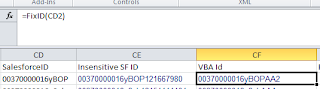## Tuesday, February 19, 2013

### Making a Salesforce Id case insensitive in Excel

I have an Excel spreadsheet with a large number of rows. Each row includes the 15 character case sensitive version of a Salesforce Id. I need to find the rows that contain duplicate Salesforce Ids.

With the 15 character Salesforce Ids being case sensitive, and Excel by and large being case insensitive this presents an issue.

## Possible Solutions

1. Export the data from Salesforce using the 18 character Salesforce Ids. These are specifically designed to be case insensitive.
2. Use an Excel formula to create another column containing a case insensitive value.
`=CD2&CODE(MID(CD2,12,1))&CODE(MID(CD2,13,1))&CODE(MID(CD2,14,1))&CODE(MID(CD2,15,1))`
Here the cell CD2 contains the case sensitive Salesforce Id.
This formula is appending the ASCII character value for each of the last 4 characters in the ID.
For example, an ID that ends with "yBSe" will have "1216683101" appended.
You may need to extend the number of checked characters if you have a large number of records. As it is only the last 4 characters of the input Id will be case checked.3. Use VBA to calculate the 18 character case insensitive values.
Place the following into a VBA module.
```Function FixID(InID As String) As String
' Converts a 15 character ID to an 18 character, case-insensitive one ...
Dim InChars As String, InI As Integer, InUpper As String
Dim InCnt As Integer
InChars = "ABCDEFGHIJKLMNOPQRSTUVWXYZ012345"
InUpper = "ABCDEFGHIJKLMNOPQRSTUVWXYZ"

InCnt = 0
For InI = 15 To 1 Step -1
InCnt = 2 * InCnt + Sgn(InStr(1, InUpper, Mid(InID, InI, 1), vbBinaryCompare))
If InI Mod 5 = 1 Then
FixID = Mid(InChars, InCnt + 1, 1) + FixID
InCnt = 0
End If
Next InI
FixID = InID + FixID
End Function
```Then reference the function directly in a formula.